【转】不同寻常的数

（转载者）【逻辑引擎】简序：虽然关于超限数的一些理论（特别是大基数）遭到某些直觉主义者或构造主义者的诟病，但对我个人而言，如果非要让我在我所有的知识中挑选出唯一一种美到令我窒息的东西，那就是超限数。这是疯子数学天才康托尔（Georg Cantor）开启的通往无穷地狱的神奇窗口。对我而言，超限数比任何疯狂的幻想小说都更加疯狂。你一旦进入了这个浩瀚的世界，就会发现自己所知道的一切，甚至是自己最为疯狂的幻想，都是无限微不足道的。相比于原则上不受任何限制可随意创造甚至连逻辑含混不清自相矛盾都可容忍的幻想小说设定，超限数却是在绝对严格地遵守最苛刻规则的同时，又绝对无限地突破任意强大的智力能够触及的极限。即便是强大到能创造整个宇宙的造物主，拥有对人类而言真正无穷大的计算能力，在超限数的层级上充其量可以比我们多走一点点。而在超限数这个浩瀚的世界中，多走这一点点只不过是无限微不足道的进展。仅凭这一点，我就愿意用一生去欣赏它。

——原来在BBS上发表的译文不支持TeX公式，许多符号只好用字符替代，我此次转载将这些符号全部替换成了TeX公式。此外，我对一些引起某些读者困惑的内容加了几条新的注释。

————————————————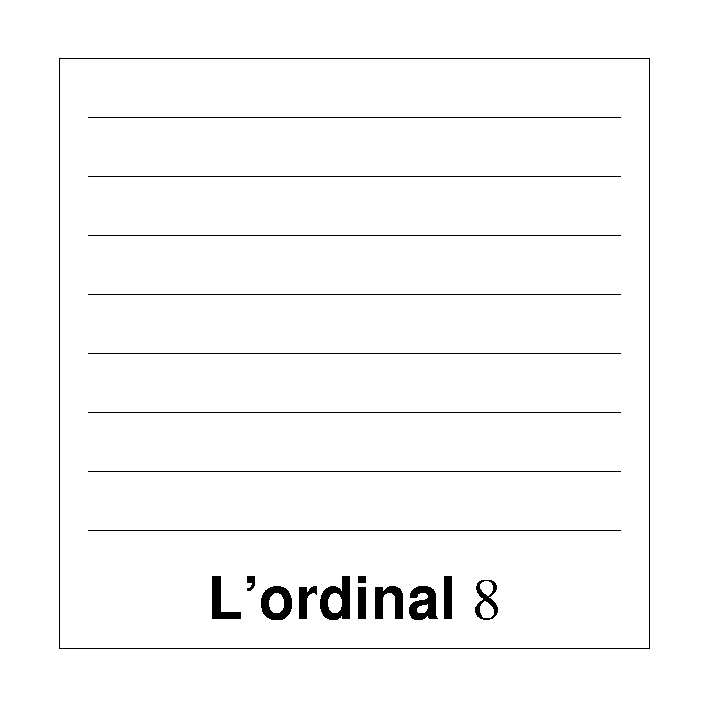（乌龟开始以一个令阿基里斯吃惊的速度朝前走——他几乎跟不上她的脚步。）

（阿基里斯吃惊地四下张望。他有点不太明白自己是怎么走到这里来的。在他的前面是一幅新的画，题目为“序数”。画的还是水平横线。不过这次这些线越往上就排得越紧，在画的顶部，线条排得密密麻麻分不清了。）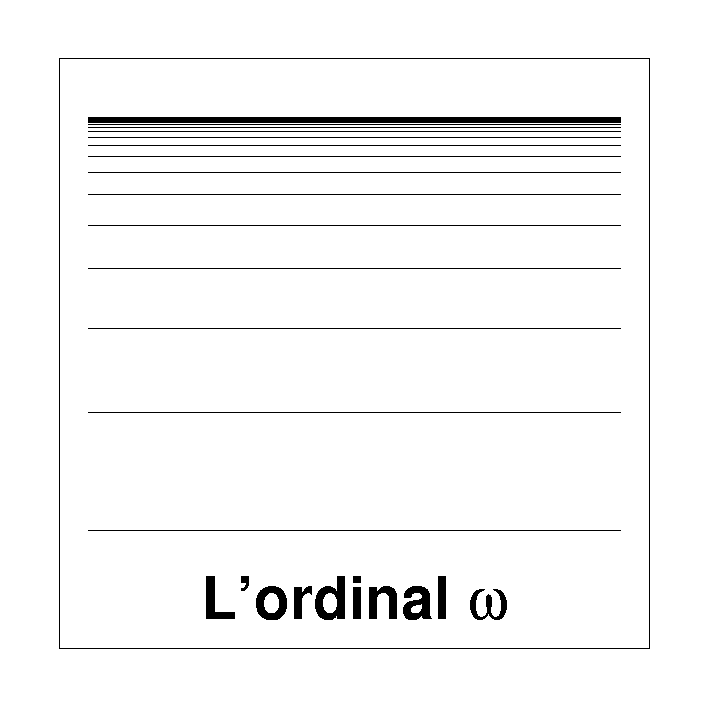（阿基里斯听话地往右看，当他瞧见那幅题为“序数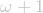”的画时，张口结舌。这是一幅和“序数”几乎一模一样的画，差别就是在最上头多了一条线。）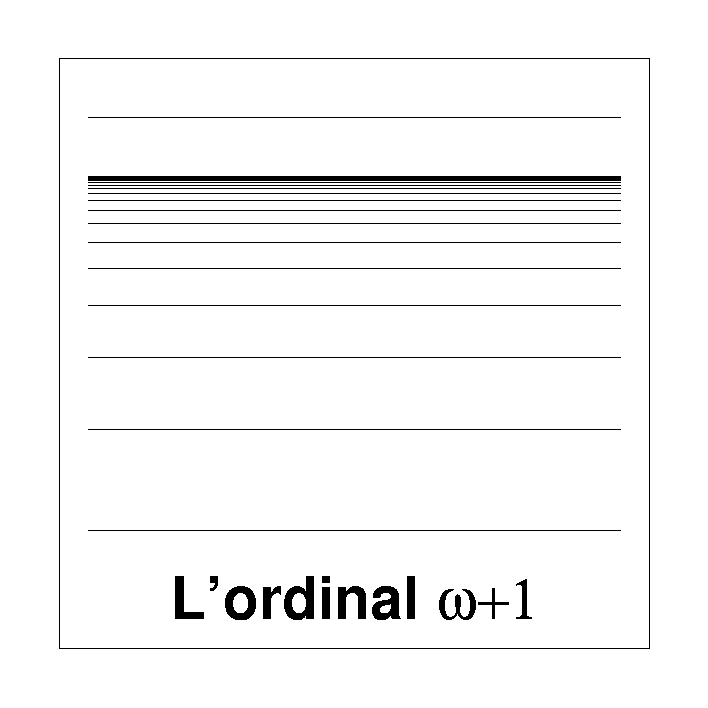（在超空间中再次小小一跃后，阿基里斯和乌龟站在了一幅题为“序数”的画前。）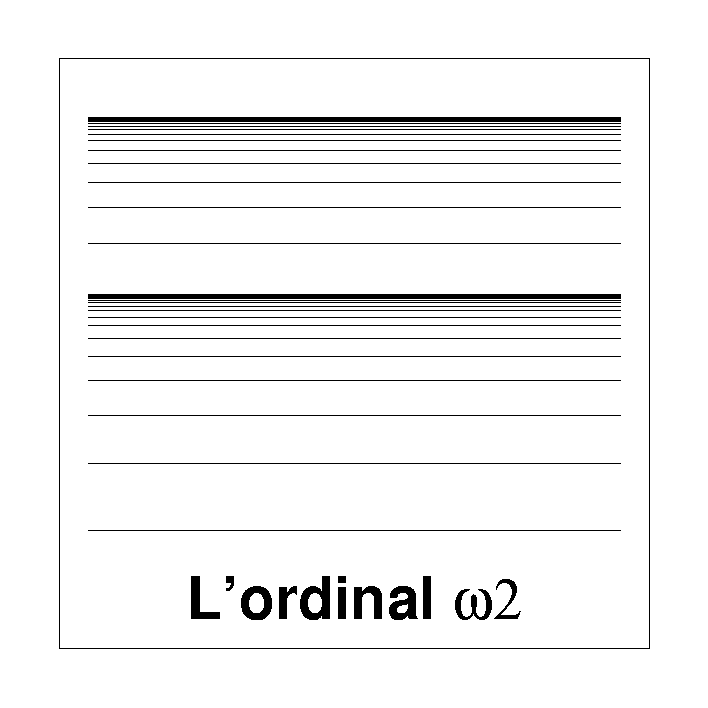（乌龟（跑够了）打了个响指（乌龟做这种事，和她能跑步一样，都很让人吃惊的），她和阿基里斯正站在一幅名叫“序数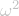”的画前。阿基里斯心悦诚服地陷入了沉思。）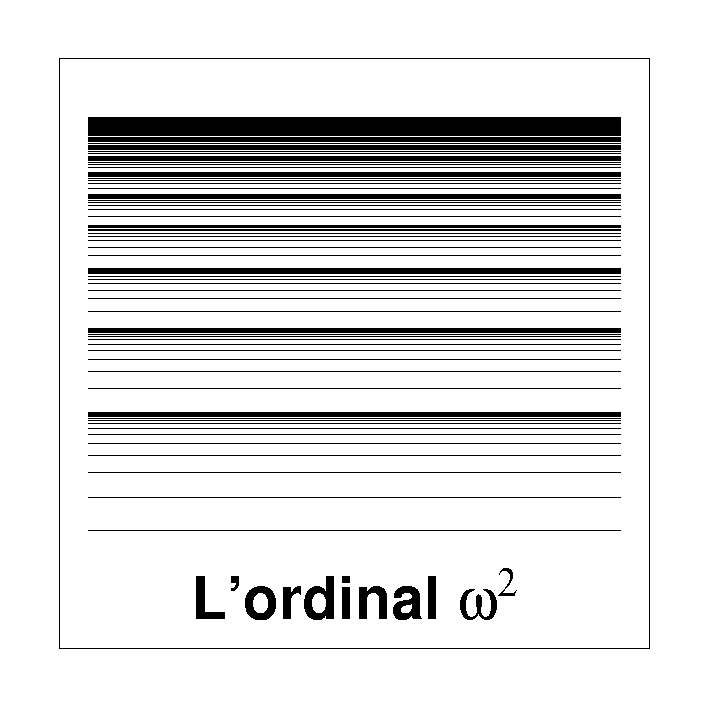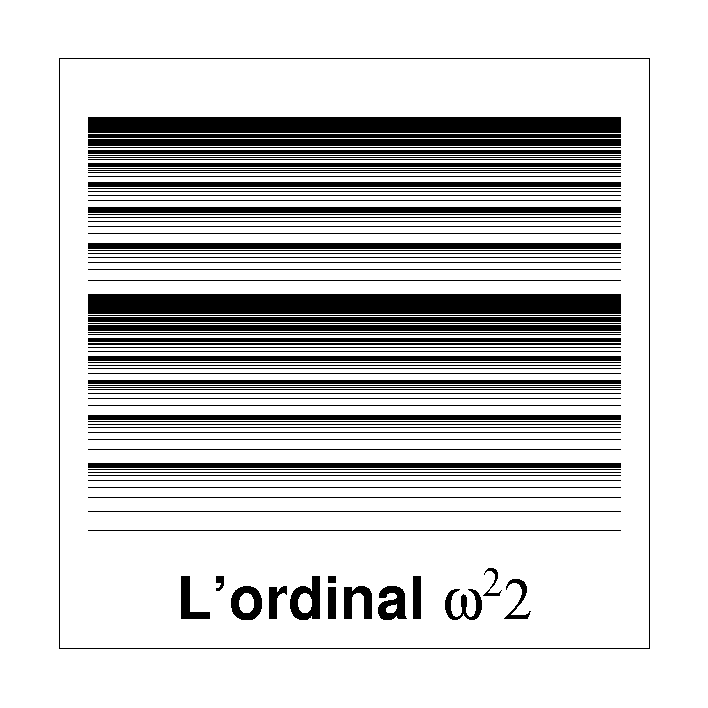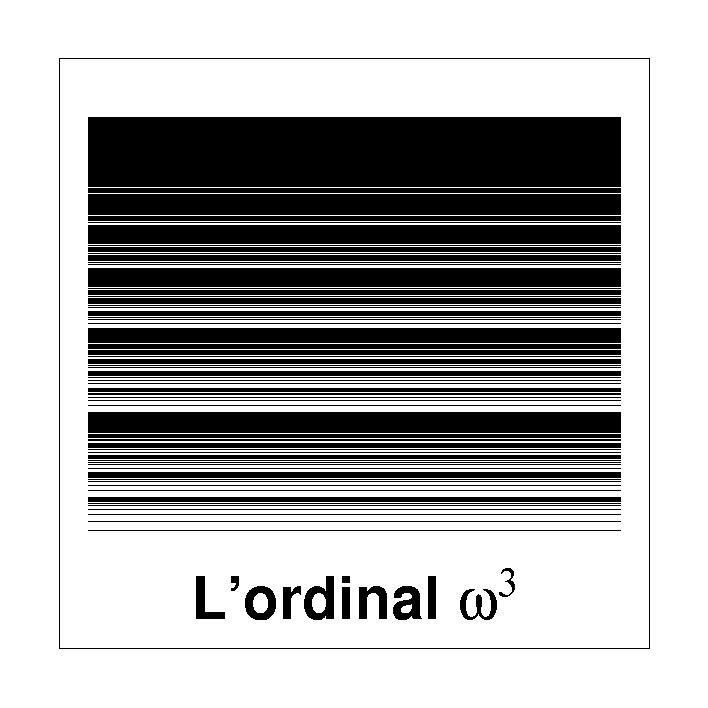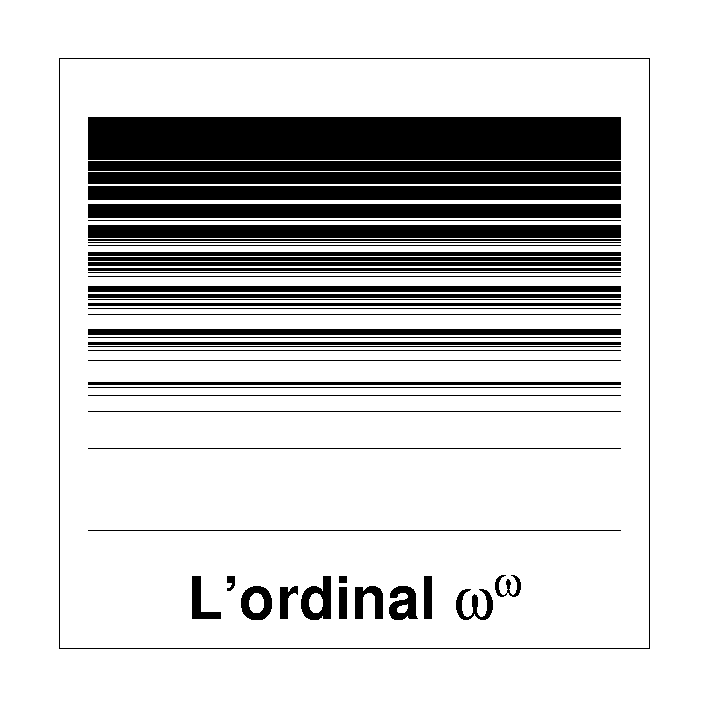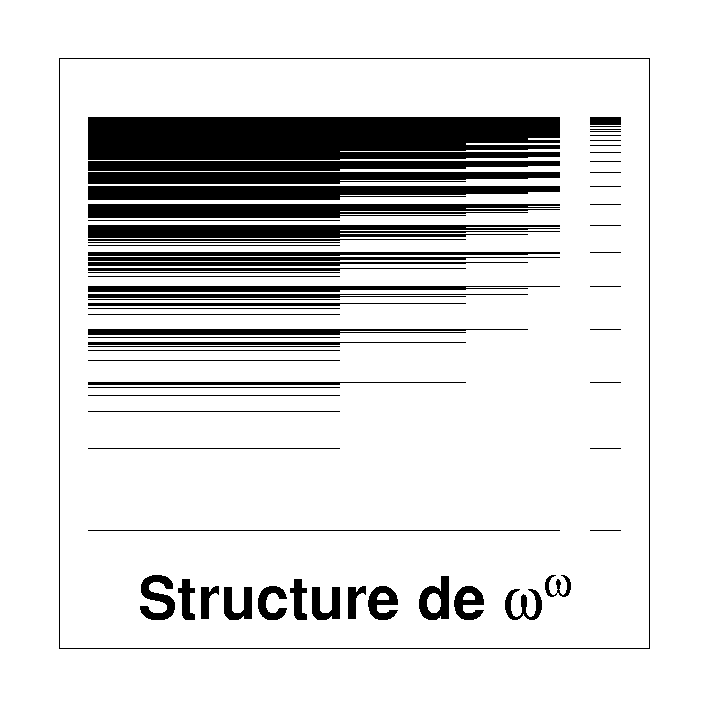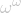的结构

（一个保安逼近。）

[注1] 原题为“Des nombres peu ordinaires”，这里ordinaire一语双关，既指“寻常”，又和“序数”(ordinal，原意为“表示顺序的”)一词相近。以阿基里斯和乌龟这两个芝诺悖论的主角的对话形式来介绍数学主题，尤其是关于无限的主题，似乎是候世达在其名著《集异壁》里的发明。在中文版《集异壁》中，乌龟是个男性的角色，因为阿基里斯总以“龟兄”来称呼，而法文版中乌龟却是女性角色，因为乌龟（La Tortue）在法文中为阴性。本文原文为法文，故译文保持乌龟的女性形象。阿基里斯又译为阿喀琉斯，是古希腊神话中马密顿国王佩琉斯和海洋女神泰提斯的儿子，半人半神的伟大英雄。

[注2] 阿列封斯·阿莱（Alphonse Allais，1855-1905），法国作家。

[注3]是希腊字母的最后一个字母，代表终结。如《新约·启示录》中说：“我是阿拉法、我是俄梅戛、我是首先的、我是末后的、我是初、我是终。”（启22:13）阿拉法即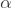，希腊字母中的第一个，俄梅戛即[注4] 德语，意为“不可企及者，在此事已成。”

[注5] 德日进（Pierre Teilhard de Chardin，1881-1955），法国思想家，地质学家和古生物学家、天主教耶稣会修士，著作中有关于无限和永恒的论述。

[注6]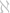是希伯莱文的第一个字母，读作“阿列夫”；就读作“阿列夫零”，其他下标也以此类推。

[注7] 这是乌龟在学神探福尔摩斯的口气半开玩笑地称赞阿基里斯。

[注8] 这里乌龟显然想起了龟兔赛跑的故事。

[注9] 原文直译是“以为自己是拿破仑”，法文俗语，即不知道自己的斤两，脑子有点疯了。这里引用电影《大腕》里的笑话翻译。

[注10] 司汤达综合症是指由于欣赏艺术作品而引起激动情绪后的身体不适，以法国作家司汤达的名字命名。

[注11] 按古希腊神话，当阿基里斯还是婴儿时，他的母亲忒提斯曾握住他的脚踵，倒提着将他在冥河水中浸泡过，使他全身刀枪不入，只有被捏住的脚踵是个例外。在特洛伊战争中，他被暗箭射中脚踵而死。所以“阿基里斯之踵”一词常被用来形容“唯一的致命处”。

[注12] 马赫洛基数以数学家Paul Mahlo的名字命名，它是Mahlo于1911年首先提出的

[【逻辑引擎】注1] 通俗地讲，你可以认为每一个序数就代表它下面按顺序排列的所有比它小的序数的序列。例如序数3就代表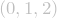，序数就代表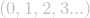，序数就代表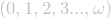[【逻辑引擎】注2] 这段话有点晦涩，我稍微解释一下。乌龟试图向阿基里斯说明，即便是看上去非常恐怖的序数里面的横档的“数量”跟自然数也是完全相等的，可以给其中每一条横档都赋予独一无二的自然数编码。具体怎样做呢？序数的画中的每一条横档对应的序数都形如：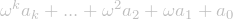，其中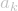是自然数，可以对每个这样的序数赋予一个独一无二的自然数编号：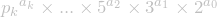，其中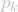是第k个素数，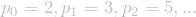。现在每一个横档都有了一个独一无二的自然数编号，跟自然数之间就建立了一一对应关系，因此也就证明了这幅画里面的横档数量仍然是可数的。

[【逻辑引擎】注3] 在通常的集合论公理系统ZFC中无法证明这么大的基数的存在性，必须引入断言大基数存在的公理。但这是否跟ZFC相容，根据维基百科直到最近（2006）也无人知道，只知道断言大基数不存在的公理跟ZFC相容。特别巨大的大基数Reinhardt cardinal，已经被证明跟ZFC+j或NBG+AC不相容，而是否能跟ZF+j或NBG相容，根据维基百科直到最近（2006）也无人知道。

【逻辑引擎】维基百科相关条目参考链接：

Ordinal number

Cardinal number

Transfinite number

Limit ordinal

Ordinal arithmetic

Cofinal

Cofinality

Large countable ordinal

Church-Kleene ordinal

First uncountable ordinal

Limit cardinal

Beth number

Regular cardinal

Large cardinal

List of large cardinal properties

Measurable cardinal

Inaccessible cardinal

Mahlo cardinal

Rank into rank

Reinhardt cardinal

Extendible cardinal

Supercompact cardinal

Reflection principle

Vopěnka’s principle

Huge cardinal

Dehornoy order

Stationary set

自然数的Peano公理系统

eq0..自反性（reflexive）
eq1..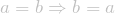对称性（symmetric）
eq2.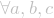.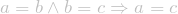传递性（transitive）
eq3.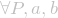.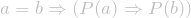相等则具有相同属性。

n0.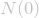0是自然数
n1..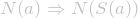自然数的后继也是自然数
n2..仅当两自然数相等后继才可能相等
n3..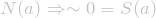0不是任何自然数的后继
n4.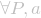.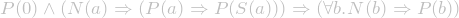归纳公理的二阶逻辑版本。对于任意属性P和任意自然数a，如果【a)0具有属性P，b)只要a具有属性P则a的后继也具有属性P】，那么任意自然数都具有属性P。如果引入集合概念就可以只用一阶逻辑。

我求得的全新Einstein场方程精确解

（孙伊2013-12-20于上海交大闵行物理楼）

US解形式如下（具体推导过程请参考我的原始论文JCAP 1101:031,2011或勘误版arXiv:1102.2609）：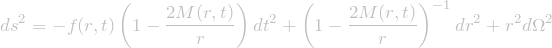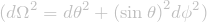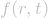是由密度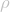和径向压强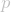决定的（注，切向压强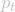不独立于而被吸收掉了）：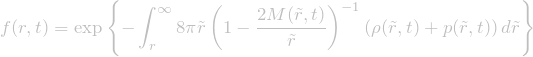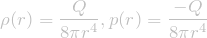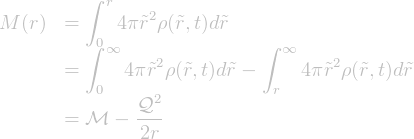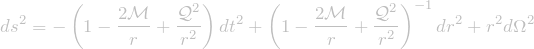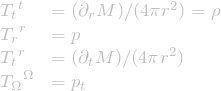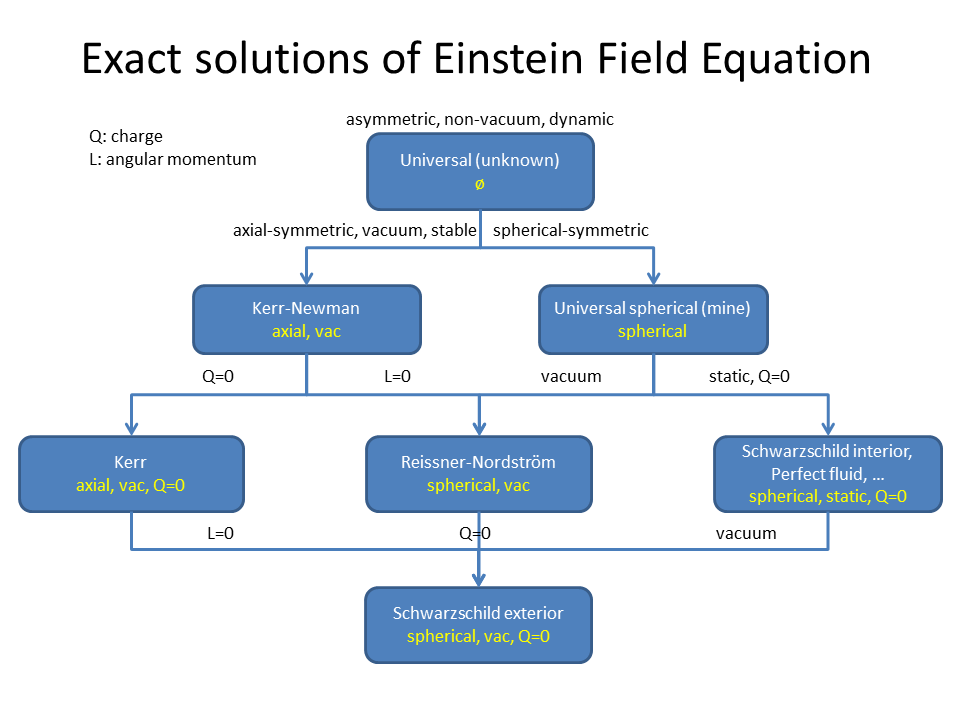解释一下扩散方程

（各向同性的）扩散方程：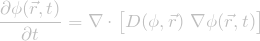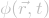是某点某时刻的浓度，是某点在浓度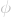下的扩散系数。如果扩散系数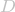是常数，那么扩散方程退化为热传导方程：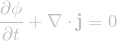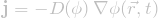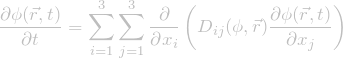对一道关于三扇门赌局的概率问题的解答

————————————————————————————

————————————————————————————

1.无论你是否选中车，他都会打开另一扇没车的门。（此时换选就是2/3得车）
2.只要你没选中车，他就会打开另一扇没车的门。（此时换选就是100%得车）
3.只要你选中了车，他就会打开另一扇门。（此时换选就必然没车）
4.以上策略的概率混合策略……（根据不同的混合，换选会有不同的概率得车）

一本好书中流露出的傲慢与偏见

————————————————————————————

————————————————————————————
（接下来是我续的一段）

————————————————————————————

【数学科普】数系为什么要一次又一次地扩充？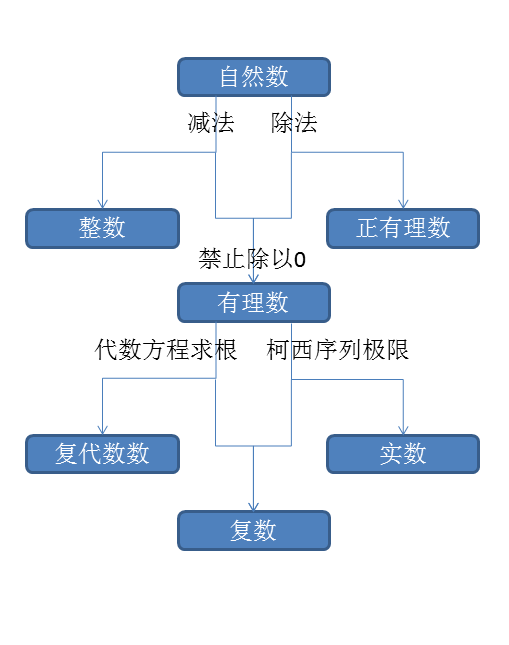又一个巨大的数学反例

Let f(n) = GCD(n^17+9, (n+1)^17+9), then f(n) = 1 for all n < = 8424432925592889329288197322308900672459420460792432 but f(8424432925592889329288197322308900672459420460792433) = 8936582237915716659950962253358945635793453256935559 > 1

形式对称的Fourier transform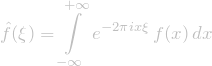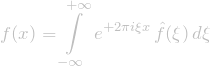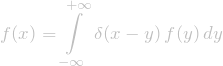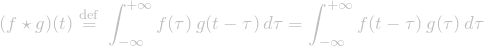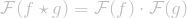Benford’s Law

Wikipedia上有这个法则的若干种解释：

<Benford’s Law>

转载，数学反例

wwwckq 发表于 2011-7-27 10:59:00

数学猜想并不总是对的，错误的数学猜想不占少数。关键在于，有时反例太大，找出反例实在是太困难了。这篇日志收集了很多大反例的例子，里面提到的规律看上去非常诱人，要试到相当大的数时才会出现第一个反例。

圆上有 n 个点，两两之间连线后，最多可以把整个圆分成多少块？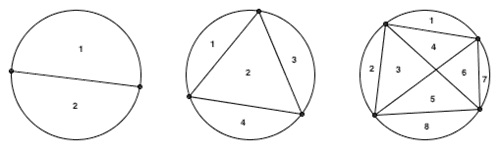上图显示的就是 n 分别为 2 3 4 的情况。可以看到，圆分别被划分成了 2 块、 4 块、 8 块。规律似乎非常明显：圆周上每多一个点，划分出来的区域数就会翻一倍。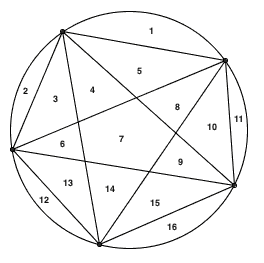果然不出所料，整个圆被分成了 16 块，区域数依旧满足 2n-1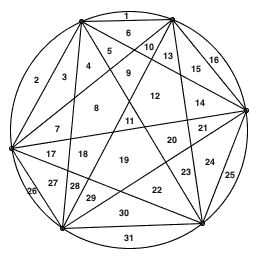此时区域数只有 31 个。

1772 年，Euler 曾经发现，当 n 是正整数时， n2 + n + 41 似乎总是素数。事实上，n 1 一直取到 39，算出来的结果分别是：

43, 47, 53, 61, 71, 83, 97, 113, 131, 151, 173, 197, 223, 251, 281,
313, 347, 383, 421, 461, 503, 547, 593, 641, 691, 743, 797, 853,
911, 971, 1033, 1097, 1163, 1231, 1301, 1373, 1447, 1523, 1601

这些数全都是素数。第一次例外发生在 n = 40 的时候，此时 402 + 40 + 41 = 402 + 40 + 40 + 1 = (40 + 1)(40 + 1) = 41 × 41

xn – 1 的因式分解

x2 – 1 分解因式后等于 (x + 1)(x – 1) x20 – 1 分解因式后等于

(x – 1) (x + 1) (x2 + 1) (x4 – x3 + x2 – x + 1) (x4 + x3 + x2 + x + 1) (x8 – x6 + x4 – x2 + 1)

对于所有的正整数 n xn – 1 因式分解后各项系数都只有可能是 1 或者 -1 吗？据说有人曾经算到了 x100 – 1 ，均没有发现反例，终于放心大胆地做出了这个猜想。悲剧的是，这个猜想是错误的，第一个反例出现在 n = 105 的情况， x105 – 1 分解出来等于

(x – 1) (x2 + x + 1) (x4 + x3 + x2 + x + 1) (x6 + x5 + x4 + x3 + x2 + x + 1)
(x8 – x7 + x5 – x4 + x3 – x + 1) (x12 – x11 + x9 – x8 + x6 – x4 + x3 – x + 1)
(x24 – x23 + x19 – x18 + x17 – x16 + x14 – x13 + x12 – x11 + x10 – x8 + x7 – x6 + x5 – x + 1)
(x48 + x47 + x46 – x43 – x422 x41 – x40 – x39 + x36 + x35 + x34 + x33 + x32 + x31 – x28
– x26 – x24 – x22 – x20 + x17 + x16 + x15 + x14 + x13 + x12 – x9 – x82 x7 – x6 – x5 + x2 + x + 1)

2 为底的伪素数

下面是当 n 较小的时候， n 2n – 2 的值。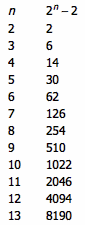似乎有这样的规律： n 能整除 2n – 2 ，当且仅当 n 是一个素数。如果真是这样的话，我们无疑有了一种超级高效的素数判定算法（ 2n

事实上，根据 Fermat 小定理，如果 p 是素数，那么 p 一定能整除 2n – 2。不过，它的逆定理却是不成立的，上面提到的 341 便是一例。我们把这种数叫做以 2 为底的伪素数。由于这种素数判定法的反例出人意料的少，我们完全可以用它来做一个概率型的素数判定算法。事实上，著名的素性测试算法就是用的这个原理。

Perrin 伪素数

定义 f(n) = f(n – 2) + f(n – 3) ，其中 f(1) = 0 f(2) = 2 f(3) = 3 。这个数列叫做 Perrin 数列。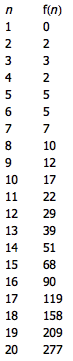似乎有这么一个规律： n 能整除 Perrin 数列的第 n f(n) ，当且仅当 n 是一个素数。如果这个规律成立的话，我们也将获得一个效率非常高的素数检验方法。根据的描述，1899 Perrin 本人曾经做过试验，随后 Malo 1900 年， Escot 1901 年，以及 Jarden 1966 年都做过搜索，均未发现任何反例。直到 1982 年， Adams Shanks 才发现第一个反例 n = 271 441 ，它等于 521 × 521 ，却也能整除 f(271 441) 。下一个反例则发生在 n = 904 631 的时候，再下一个反例则是 n = 16 532 714 。这种反例被称为 Perrin 伪素数。

说到大反例，这是我最喜欢举的例子。下面是大于 1 的正整数分解质因数后的结果：

2 = 2
3 = 3
4 = 2 × 2
5 = 5
6 = 2 × 3
7 = 7
8 = 2 × 2 × 2
9 = 3 × 3
10 = 2 × 5

其中，46910 包含偶数个质因子，其余的数都包含奇数个质因子。你会发现，在上面的列表中一行一行地看下来，不管看到什么位置，包含奇数个质因子的数都要多一些。 1919 年，George Pólya 猜想，质因子个数为奇数的情况不会少于 50% 。也就是说，对于任意一个大于 1 的自然数 n ，从 2 n 的数中有奇数个质因子的数不少于有偶数个质因子的数。这便是著名的 Pólya 猜想。

Pólya 猜想看上去非常合理——每个有偶数个质因子的数，必然都已经提前经历过了有奇数个质因子这一步。不过，这个猜想却一直未能得到一个严格的数学证明。到
1958 年，英国数学家 C. B. Haselgrove 发现， Pólya 猜想竟然是错误的。他证明了 Pólya 猜想存在反例，从而推翻了这个猜想。不过，Haselgrove 仅仅是证明了反例的存在性，并没有算出这个反例的具体值。Haselgrove 估计，这个反例至少也是一个 361 位数。

1960 年，R. Sherman Lehman 给出了一个确凿的反例：n = 906 180 359。而 Pólya 猜想的最小反例则是到了 1980 年才发现的：n = 906 150 257

Fermat 大定理还能推广吗？

Fermat 大定理说，当 n > 2 时，方程 xn + yn = zn

XX 型平方数

11, 22, 33, 44, 55, 66, 77, 88, 99, 1010, 1111, 1212, … 都不是完全平方数。有没有什么数，把它连写两次后，正好是一个完全平方数呢？有。第一个这样的数是 13 223 140 496 ，把它连写两次将得到 1 322 314 049 613 223 140 496 ，是 36 363 636 364 的平方。第二个这样的数则是 20 661 157 025 ，它对应了 45 454 545 455 的平方。更多信息可见

下面是 n 为正整数时， 2 / (21/n – 1) 取上整的结果与 2n / ln(2) 取下整的结果：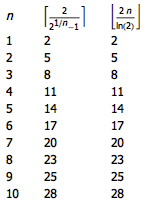这两者的结果总是相等吗？不是的。第一个反例是 n = 777 451 915 729 368，前者算出来的结果是 2 243 252 046 704 767 ，但后者是 2 243 252 046 704 766 。下一个反例则出现在 n = 140 894 092 055 857 794 的时候。更多信息可见

有没有什么猜想，明明已经被推翻了，所有人都知道存在反例，但因为反例实在是太大了，直到现在仍然没有找到呢？有。下面这张表展示了 n 取不同值时 pi(n) li(n) 的值，其中 pi(n) 表示不超过 n 的素数的个数，li(n) 则是对数积分0n dx/ln(x)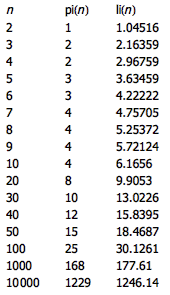pi(n) 是否永远小于 li(n) 呢？1914 年，Littlewood 证明了存在一个大数 n 使得 pi(n) ≥ li(n) ，不过却并没有给出一个具体的 n 值来。1955 年，Skewes 给出了这样的 n 值的一个上界：在 10^(10^(10^963)) 以内，必有一个满足 pi(n) ≥ li(n) n

虽然数学家们正在不断地改进上界（目前的上界大约是 e727.9513
），但仍然无法找出一个具体的 n 来。原因很简单——这个反例实在是太大了。

连转带评：纽科姆悖论

M：一天，一个由外层空间来的超级生物欧米加在地球着陆。

M：欧米加搞出一个设备来研究人的大脑。他可以十分准确地预言每一个人在二者择一时会选择哪一个。

M：欧米加用两个大箱子检验了很多人。箱子A是透明的，总是装着1千美元。箱子B不透明，它要么装着1百万美元，要么空着。

M：欧米加告诉每一个受试者。

M：这个男人决定只拿箱子B。他的理由是——

M：这个女孩决定要拿两个箱子，她的理由是——

M：你认为谁的决定最好？两种看法不可能都对。哪一种错了？它为何错了？这是一个新的悖论，而专家们还不知道如何解决它。

——————————————————————————————

Zorn’s Lemma

Suppose a partially ordered set has the property that every chain (i.e. totally ordered subset) has an upper bound. Then the set contains at least one maximal element.

Zorn’s lemma is equivalent to the well-ordering theorem and the axiom of choice, in the sense that any one of them, together with the Zermelo–Fraenkel axioms of set theory, is sufficient to prove the others.

Zorn’s lemma is equivalent (in ZF) to three main results:

Hausdorff maximal principle
Axiom of choice
Well-ordering theorem

Moreover, Zorn’s lemma (or one of its equivalent forms) implies some major results in other mathematical areas. For example,

Banach’s extension theorem which is used to prove one of the most fundamental results in functional analysis, the Hahn–Banach theorem
Every vector space has a Hamel basis, a result from linear algebra
Every commutative unital ring has a maximal ideal, a result from ring theory
Tychonoff’s theorem in topology

In this sense, we see how Zorn’s lemma can be seen as a powerful tool, especially in the sense of unified mathematics.

Finding all paths from source to destination

 123456789101112131415161718 stack node_stack; set node_set; AllPathFromSourceToDestination(node s, node d) {     node_stack.push(s);     node_set.add(s); //mark each node in the path     // for each node v that adjacent with node s:     for_each(node v, adjacent(v,s)) {         if(v == d) {             Output(node_stack);         } else {             if(node_set.has(v)==false) {                 AllPathFromSourceToDestination(v, d);             }         }     }     node_set.del(s);     node_stack.pop(s); }

Sorting network

The following is network-sorting algorithm for less than 33 elements.

What is Sorting Network? Wiki: sorting network

List of sorting networks:
From: sorting networks
Input the number n (maximum 32)
Algorithm choices: “Best”
Click [Take a look] button.

—————————
Zero-one principle

While it is easy to prove the validity of some sorting networks (like the insertion/bubble sorter), it is not always so easy. There are n! permutations of numbers in an n-wire network, and to test all of them would take a significant amount of time, especially when they are larger. However, because of the so-called zero-one principle, far fewer trials are in fact needed to test the validity of a sorting network.

The zero-one principle states that a sorting network is valid if it can sort all 2n sequences of 0s and 1s. This not only drastically cuts down on the number of tests needed to ascertain the validity of a network, it is of great use in creating many constructions of sorting networks as well. The principle has been proven by a special case of the Bouricius’s Theorem in 1954 by W. G. Bouricius.

 123456789101112131415161718192021222324252627282930313233343536373839404142434445464748495051525354555657585960616263646566676869707172737475767778798081828384858687888990919293949596979899100101102103104105106107108109110111112113114115116117118119120121122123124125126127128129130131132133134135136137138139140141142143144145146147148149150151152153154155156157158159160161162163164165166167168169170171172173174175176177178179180181182183184185186187188189190191192193194195196197198199200201202203204205206207208209210211212213214215216217218219220221222223224225226227228229230231232233234235236237238239240241242243244245246247248249250251252253254255256257258259260261262263264265266267268269270271272273274275276277278279280281282283284285286287288289290291292293294295296297298299300301302303304305306307308309310311312313314315316317318319320321322323324325326327328329330331332333334335336337338339340341342343344345346347348349350351352353354355356357358359360361362363364365366367368369370371372373374375376377378379380381382383384385386387388389390391392393394395396397398399400401402403404405406407408409410411412413414415416417418419420421422423424425426427428429430431432433434435436437438439440441442443444445446447448449450451452453454455456457458459460461462463464465466467468469470471472473474475476477478479480481482483484485486487488489490491492493494495496497498499500501502503504505506507508509510511512513514515516517518519520521522523524525526527528529530531532533534535536537538539540541542543544545546547548549550551552553554555556557558559560561562563564565566567568569570571572573574575576577578579580581582583584585586587588589590591592593594595596597598599600601602603604605606607608609610611612613614615616617618619620621622623624625626627628629630631632633634635636637638639640 static const unsigned int network02[] = {     { 0, 1}, }; static const unsigned int network03[] = {     { 1, 2},     { 0, 2},     { 0, 1}, }; static const unsigned int network04[] = {     { 0, 1}, { 2, 3},     { 0, 2}, { 1, 3},     { 1, 2}, }; static const unsigned int network05[] = {     { 0, 1}, { 3, 4},     { 2, 4},     { 2, 3}, { 1, 4},     { 0, 3},     { 0, 2}, { 1, 3},     { 1, 2}, }; static const unsigned int network06[] = {     { 1, 2}, { 4, 5},     { 0, 2}, { 3, 5},     { 0, 1}, { 3, 4}, { 2, 5},     { 0, 3}, { 1, 4},     { 2, 4}, { 1, 3},     { 2, 3}, }; static const unsigned int network07[] = {     { 1, 2}, { 3, 4}, { 5, 6},     { 0, 2}, { 3, 5}, { 4, 6},     { 0, 1}, { 4, 5}, { 2, 6},     { 0, 4}, { 1, 5},     { 0, 3}, { 2, 5},     { 1, 3}, { 2, 4},     { 2, 3}, }; static const unsigned int network08[] = {     { 0, 1}, { 2, 3}, { 4, 5}, { 6, 7},     { 0, 2}, { 1, 3}, { 4, 6}, { 5, 7},     { 1, 2}, { 5, 6}, { 0, 4}, { 3, 7},     { 1, 5}, { 2, 6},     { 1, 4}, { 3, 6},     { 2, 4}, { 3, 5},     { 3, 4}, }; static const unsigned int network09[] = {     { 0, 1}, { 3, 4}, { 6, 7},     { 1, 2}, { 4, 5}, { 7, 8},     { 0, 1}, { 3, 4}, { 6, 7}, { 2, 5},     { 0, 3}, { 1, 4}, { 5, 8},     { 3, 6}, { 4, 7}, { 2, 5},     { 0, 3}, { 1, 4}, { 5, 7}, { 2, 6},     { 1, 3}, { 4, 6},     { 2, 4}, { 5, 6},     { 2, 3}, }; static const unsigned int network10[] = {     { 4, 9}, { 3, 8}, { 2, 7}, { 1, 6}, { 0, 5},     { 1, 4}, { 6, 9}, { 0, 3}, { 5, 8},     { 0, 2}, { 3, 6}, { 7, 9},     { 0, 1}, { 2, 4}, { 5, 7}, { 8, 9},     { 1, 2}, { 4, 6}, { 7, 8}, { 3, 5},     { 2, 5}, { 6, 8}, { 1, 3}, { 4, 7},     { 2, 3}, { 6, 7},     { 3, 4}, { 5, 6},     { 4, 5}, }; static const unsigned int network11[] = {     { 0, 1}, { 2, 3}, { 4, 5}, { 6, 7}, { 8, 9},     { 1, 3}, { 5, 7}, { 0, 2}, { 4, 6}, { 8,10},     { 1, 2}, { 5, 6}, { 9,10}, { 0, 4}, { 3, 7},     { 1, 5}, { 6,10}, { 4, 8},     { 5, 9}, { 2, 6}, { 0, 4}, { 3, 8},     { 1, 5}, { 6,10}, { 2, 3}, { 8, 9},     { 1, 4}, { 7,10}, { 3, 5}, { 6, 8},     { 2, 4}, { 7, 9}, { 5, 6},     { 3, 4}, { 7, 8}, }; static const unsigned int network12[] = {     { 0, 1}, { 2, 3}, { 4, 5}, { 6, 7}, { 8, 9}, {10,11},     { 1, 3}, { 5, 7}, { 9,11}, { 0, 2}, { 4, 6}, { 8,10},     { 1, 2}, { 5, 6}, { 9,10}, { 0, 4}, { 7,11},     { 1, 5}, { 6,10}, { 3, 7}, { 4, 8},     { 5, 9}, { 2, 6}, { 0, 4}, { 7,11}, { 3, 8},     { 1, 5}, { 6,10}, { 2, 3}, { 8, 9},     { 1, 4}, { 7,10}, { 3, 5}, { 6, 8},     { 2, 4}, { 7, 9}, { 5, 6},     { 3, 4}, { 7, 8}, }; static const unsigned int network13[] = {     { 1, 7}, { 9,11}, { 3, 4}, { 5, 8}, { 0,12}, { 2, 6},     { 0, 1}, { 2, 3}, { 4, 6}, { 8,11}, { 7,12}, { 5, 9},     { 0, 2}, { 3, 7}, {10,11}, { 1, 4}, { 6,12},     { 7, 8}, {11,12}, { 4, 9}, { 6,10},     { 3, 4}, { 5, 6}, { 8, 9}, {10,11}, { 1, 7},     { 2, 6}, { 9,11}, { 1, 3}, { 4, 7}, { 8,10}, { 0, 5},     { 2, 5}, { 6, 8}, { 9,10},     { 1, 2}, { 3, 5}, { 7, 8}, { 4, 6},     { 2, 3}, { 4, 5}, { 6, 7}, { 8, 9},     { 3, 4}, { 5, 6}, }; static const unsigned int network14[] = {     { 0, 1}, { 2, 3}, { 4, 5}, { 6, 7}, { 8, 9}, {10,11}, {12,13},     { 0, 2}, { 4, 6}, { 8,10}, { 1, 3}, { 5, 7}, { 9,11},     { 0, 4}, { 8,12}, { 1, 5}, { 9,13}, { 2, 6}, { 3, 7},     { 0, 8}, { 1, 9}, { 2,10}, { 3,11}, { 4,12}, { 5,13},     { 5,10}, { 6, 9}, { 3,12}, { 7,11}, { 1, 2}, { 4, 8},     { 1, 4}, { 7,13}, { 2, 8}, { 5, 6}, { 9,10},     { 2, 4}, {11,13}, { 3, 8}, { 7,12},     { 6, 8}, {10,12}, { 3, 5}, { 7, 9},     { 3, 4}, { 5, 6}, { 7, 8}, { 9,10}, {11,12},     { 6, 7}, { 8, 9}, }; static const unsigned int network15[] = {     { 0, 1}, { 2, 3}, { 4, 5}, { 6, 7}, { 8, 9}, {10,11}, {12,13},     { 0, 2}, { 4, 6}, { 8,10}, {12,14}, { 1, 3}, { 5, 7}, { 9,11},     { 0, 4}, { 8,12}, { 1, 5}, { 9,13}, { 2, 6}, {10,14}, { 3, 7},     { 0, 8}, { 1, 9}, { 2,10}, { 3,11}, { 4,12}, { 5,13}, { 6,14},     { 5,10}, { 6, 9}, { 3,12}, {13,14}, { 7,11}, { 1, 2}, { 4, 8},     { 1, 4}, { 7,13}, { 2, 8}, {11,14}, { 5, 6}, { 9,10},     { 2, 4}, {11,13}, { 3, 8}, { 7,12},     { 6, 8}, {10,12}, { 3, 5}, { 7, 9},     { 3, 4}, { 5, 6}, { 7, 8}, { 9,10}, {11,12},     { 6, 7}, { 8, 9}, }; static const unsigned int network16[] = {     { 0, 1}, { 2, 3}, { 4, 5}, { 6, 7}, { 8, 9}, {10,11}, {12,13}, {14,15},     { 0, 2}, { 4, 6}, { 8,10}, {12,14}, { 1, 3}, { 5, 7}, { 9,11}, {13,15},     { 0, 4}, { 8,12}, { 1, 5}, { 9,13}, { 2, 6}, {10,14}, { 3, 7}, {11,15},     { 0, 8}, { 1, 9}, { 2,10}, { 3,11}, { 4,12}, { 5,13}, { 6,14}, { 7,15},     { 5,10}, { 6, 9}, { 3,12}, {13,14}, { 7,11}, { 1, 2}, { 4, 8},     { 1, 4}, { 7,13}, { 2, 8}, {11,14}, { 5, 6}, { 9,10},     { 2, 4}, {11,13}, { 3, 8}, { 7,12},     { 6, 8}, {10,12}, { 3, 5}, { 7, 9},     { 3, 4}, { 5, 6}, { 7, 8}, { 9,10}, {11,12},     { 6, 7}, { 8, 9}, }; static const unsigned int network17[] = {     { 0, 1}, { 2, 3}, { 4, 5}, { 6, 7}, { 8, 9}, {10,11}, {12,13}, {15,16},     { 0, 2}, { 1, 3}, { 4, 6}, { 5, 7}, { 8,10}, { 9,11}, {14,16},     { 1, 2}, { 5, 6}, { 0, 4}, { 3, 7}, { 9,10}, {14,15}, {13,16},     { 1, 5}, { 2, 6}, {12,15}, {11,16},     { 1, 4}, { 3, 6}, {12,14}, {13,15}, { 7,16},     { 2, 4}, { 3, 5}, {13,14}, {10,15},     { 3, 4}, { 8,13}, { 9,14}, {11,15},     { 8,12}, { 9,13}, {11,14}, { 6,15},     { 9,12}, {10,13}, { 5,14}, { 7,15},     {10,12}, {11,13}, { 0, 9}, { 7,14},     {11,12}, { 0, 8}, { 1,10}, { 4,13},     { 1, 9}, { 2,11}, { 3,12}, { 5,13},     { 1, 8}, { 3,11}, { 2, 9}, { 6,13},     { 2, 8}, { 3,10}, { 7,13}, { 6,11},     { 3, 9}, { 5,10}, { 7,12},     { 3, 8}, { 4, 9}, { 7,11},     { 4, 8}, { 5, 9}, { 7,10},     { 5, 8}, { 6, 9},     { 6, 8}, { 7, 9},     { 7, 8}, }; static const unsigned int network18[] = {     { 0, 1}, { 2, 3}, { 4, 5}, { 7, 8}, { 9,10}, {11,12}, {13,14}, {16,17},     { 0, 2}, { 1, 3}, { 6, 8}, { 9,11}, {10,12}, {15,17},     { 1, 2}, { 6, 7}, { 5, 8}, {10,11}, {15,16}, {14,17},     { 4, 7}, { 3, 8}, {13,16}, {12,17},     { 4, 6}, { 5, 7}, {13,15}, {14,16}, { 8,17},     { 5, 6}, { 2, 7}, {14,15}, {11,16},     { 0, 5}, { 1, 6}, { 3, 7}, { 9,14}, {10,15}, {12,16},     { 0, 4}, { 1, 5}, { 3, 6}, { 9,13}, {10,14}, {12,15}, { 7,16},     { 1, 4}, { 2, 5}, {10,13}, {11,14}, { 0, 9}, { 6,15}, { 8,16},     { 2, 4}, { 3, 5}, {11,13}, {12,14}, { 1,10}, { 7,15},     { 3, 4}, {12,13}, { 1, 9}, { 2,11}, { 5,14}, { 8,15},     { 3,12}, { 2, 9}, { 4,13}, { 7,14},     { 3,11}, { 5,13}, { 8,14},     { 3,10}, { 6,13},     { 3, 9}, { 7,13}, { 5,10}, { 6,11},     { 8,13}, { 4, 9}, { 7,12},     { 5, 9}, { 8,12}, { 7,11},     { 8,11}, { 6, 9}, { 7,10},     { 8,10}, { 7, 9},     { 8, 9}, }; static const unsigned int network19[] = {     { 0, 1}, { 2, 3}, { 4, 5}, { 7, 8}, { 9,10}, {12,13}, {14,15}, {17,18},     { 0, 2}, { 1, 3}, { 6, 8}, {11,13}, {16,18},     { 1, 2}, { 6, 7}, { 5, 8}, {11,12}, {10,13}, {16,17}, {15,18},     { 4, 7}, { 3, 8}, { 9,12}, {14,17}, {13,18},     { 4, 6}, { 5, 7}, { 9,11}, {10,12}, {14,16}, {15,17}, { 8,18},     { 5, 6}, { 2, 7}, {10,11}, {15,16}, { 9,14}, {12,17},     { 0, 5}, { 1, 6}, { 3, 7}, {10,15}, {11,16}, {13,17},     { 0, 4}, { 1, 5}, { 3, 6}, {10,14}, {12,16}, { 7,17},     { 1, 4}, { 2, 5}, {13,16}, {11,14}, {12,15}, { 0,10}, { 8,17},     { 2, 4}, { 3, 5}, {13,15}, {12,14}, { 0, 9}, { 1,11}, { 6,16},     { 3, 4}, {13,14}, { 1,10}, { 2,12}, { 5,15}, { 7,16},     { 1, 9}, { 3,13}, { 2,10}, { 4,14}, { 8,16}, { 7,15},     { 3,12}, { 2, 9}, { 5,14}, { 8,15},     { 3,11}, { 6,14},     { 3,10}, { 7,14}, { 6,11},     { 3, 9}, { 8,14}, { 5,10}, { 7,12},     { 4, 9}, { 8,13}, { 7,11},     { 5, 9}, { 8,12}, { 7,10},     { 8,11}, { 6, 9},     { 8,10}, { 7, 9},     { 8, 9}, }; static const unsigned int network20[] = {     { 0, 1}, { 3, 4}, { 5, 6}, { 8, 9}, {10,11}, {13,14}, {15,16}, {18,19},     { 2, 4}, { 7, 9}, {12,14}, {17,19},     { 2, 3}, { 1, 4}, { 7, 8}, { 6, 9}, {12,13}, {11,14}, {17,18}, {16,19},     { 0, 3}, { 5, 8}, { 4, 9}, {10,13}, {15,18}, {14,19},     { 0, 2}, { 1, 3}, { 5, 7}, { 6, 8}, {10,12}, {11,13}, {15,17}, {16,18}, { 9,19},     { 1, 2}, { 6, 7}, { 0, 5}, { 3, 8}, {11,12}, {16,17}, {10,15}, {13,18},     { 1, 6}, { 2, 7}, { 4, 8}, {11,16}, {12,17}, {14,18}, { 0,10},     { 1, 5}, { 3, 7}, {11,15}, {13,17}, { 8,18},     { 4, 7}, { 2, 5}, { 3, 6}, {14,17}, {12,15}, {13,16}, { 1,11}, { 9,18},     { 4, 6}, { 3, 5}, {14,16}, {13,15}, { 1,10}, { 2,12}, { 7,17},     { 4, 5}, {14,15}, { 3,13}, { 2,10}, { 6,16}, { 8,17},     { 4,14}, { 3,12}, { 5,15}, { 9,17}, { 8,16},     { 4,13}, { 3,11}, { 6,15}, { 9,16},     { 4,12}, { 3,10}, { 7,15},     { 4,11}, { 8,15}, { 7,12},     { 4,10}, { 9,15}, { 6,11}, { 8,13},     { 5,10}, { 9,14}, { 8,12},     { 6,10}, { 9,13}, { 8,11},     { 9,12}, { 7,10},     { 9,11}, { 8,10},     { 9,10}, }; static const unsigned int network21[] = {     { 0, 1}, { 3, 4}, { 5, 6}, { 8, 9}, {10,11}, {13,14}, {16,17}, {19,20},     { 2, 4}, { 7, 9}, {12,14}, {15,17}, {18,20},     { 2, 3}, { 1, 4}, { 7, 8}, { 6, 9}, {12,13}, {11,14}, {15,16}, {18,19}, {17,20},     { 0, 3}, { 5, 8}, { 4, 9}, {10,13}, {15,18}, {16,19}, {14,20},     { 0, 2}, { 1, 3}, { 5, 7}, { 6, 8}, {10,12}, {11,13}, {17,19}, {16,18}, { 9,20},     { 1, 2}, { 6, 7}, { 0, 5}, { 3, 8}, {11,12}, {17,18}, {10,16}, {13,19},     { 1, 6}, { 2, 7}, { 4, 8}, {10,15}, {11,17}, {12,18}, {14,19},     { 1, 5}, { 3, 7}, {11,16}, {13,18}, { 8,19},     { 4, 7}, { 2, 5}, { 3, 6}, {11,15}, {14,18}, {13,16}, { 9,19},     { 4, 6}, { 3, 5}, {12,15}, {14,17}, { 0,11}, { 7,18},     { 4, 5}, {14,16}, {13,15}, { 0,10}, { 1,12}, { 6,17}, { 8,18},     {14,15}, { 1,11}, { 2,13}, { 5,16}, { 9,18}, { 8,17},     { 1,10}, { 3,14}, { 4,15}, { 6,16}, { 9,17},     { 4,14}, { 3,13}, { 2,10}, { 7,16},     { 4,13}, { 3,11}, { 8,16},     { 4,12}, { 3,10}, { 9,16}, { 7,13}, { 8,14},     { 4,11}, { 6,12}, { 9,15}, { 8,13},     { 4,10}, { 5,11}, { 9,14},     { 5,10}, { 6,11}, { 9,13},     { 6,10}, { 8,11}, { 9,12},     { 7,10}, { 9,11},     { 8,10},     { 9,10}, }; static const unsigned int network22[] = {     { 0, 1}, { 3, 4}, { 6, 7}, { 9,10}, {11,12}, {14,15}, {17,18}, {20,21},     { 2, 4}, { 5, 7}, { 8,10}, {13,15}, {16,18}, {19,21},     { 2, 3}, { 1, 4}, { 5, 6}, { 8, 9}, { 7,10}, {13,14}, {12,15}, {16,17}, {19,20}, {18,21},     { 0, 3}, { 5, 8}, { 6, 9}, { 4,10}, {11,14}, {16,19}, {17,20}, {15,21},     { 0, 2}, { 1, 3}, { 7, 9}, { 6, 8}, {11,13}, {12,14}, {18,20}, {17,19}, {10,21},     { 1, 2}, { 7, 8}, { 0, 6}, { 3, 9}, {12,13}, {18,19}, {11,17}, {14,20},     { 0, 5}, { 1, 7}, { 2, 8}, { 4, 9}, {11,16}, {12,18}, {13,19}, {15,20},     { 1, 6}, { 3, 8}, {12,17}, {14,19}, { 0,11}, { 9,20},     { 1, 5}, { 4, 8}, { 3, 6}, {12,16}, {15,19}, {14,17}, {10,20},     { 2, 5}, { 4, 7}, {13,16}, {15,18}, { 1,12}, { 8,19},     { 4, 6}, { 3, 5}, {15,17}, {14,16}, { 1,11}, { 2,13}, { 7,18}, { 9,19},     { 4, 5}, {15,16}, { 3,14}, { 2,11}, { 6,17}, {10,19},     { 4,15}, { 3,13}, { 5,16}, { 7,17}, {10,18},     { 4,14}, { 3,12}, { 6,16}, { 9,17},     { 4,13}, { 3,11}, { 7,16}, {10,17},     { 4,12}, { 8,16}, { 7,13},     { 4,11}, { 9,16}, { 6,12}, { 8,14},     {10,16}, { 5,11}, { 7,12}, { 9,15},     { 6,11}, {10,15}, { 9,14},     { 7,11}, {10,14}, { 9,12},     { 8,11}, {10,13},     {10,12}, { 9,11},     {10,11}, }; static const unsigned int network23[] = {     { 0, 1}, { 3, 4}, { 6, 7}, { 9,10}, {12,13}, {15,16}, {18,19}, {21,22},     { 2, 4}, { 5, 7}, { 8,10}, {11,13}, {14,16}, {17,19}, {20,22},     { 2, 3}, { 1, 4}, { 5, 6}, { 8, 9}, { 7,10}, {11,12}, {14,15}, {13,16}, {17,18}, {20,21}, {19,22},     { 0, 3}, { 5, 8}, { 6, 9}, { 4,10}, {11,14}, {12,15}, {17,20}, {18,21}, {16,22},     { 0, 2}, { 1, 3}, { 7, 9}, { 6, 8}, {13,15}, {12,14}, {19,21}, {18,20}, {11,17}, {10,22},     { 1, 2}, { 7, 8}, { 0, 6}, { 3, 9}, {13,14}, {19,20}, {12,18}, {15,21},     { 0, 5}, { 1, 7}, { 2, 8}, { 4, 9}, {13,19}, {12,17}, {14,20}, {16,21},     { 1, 6}, { 3, 8}, {13,18}, {15,20}, { 0,12}, { 9,21},     { 1, 5}, { 4, 8}, { 3, 6}, {13,17}, {16,20}, {15,18}, { 0,11}, {10,21},     { 2, 5}, { 4, 7}, {14,17}, {16,19}, { 1,13}, { 8,20},     { 4, 6}, { 3, 5}, {16,18}, {15,17}, { 1,12}, { 2,14}, { 7,19}, { 9,20},     { 4, 5}, {16,17}, { 1,11}, { 3,15}, { 6,18}, {10,20},     { 4,16}, { 3,14}, { 2,11}, { 5,17}, { 7,18}, {10,19},     { 4,15}, { 3,12}, { 6,17}, { 9,18},     { 4,14}, { 3,11}, { 7,17}, {10,18},     { 4,13}, { 8,17},     { 4,12}, { 9,17}, { 7,13}, { 8,14},     { 4,11}, {10,17}, { 6,12}, { 9,15},     { 5,11}, { 7,12}, {10,16}, { 9,14},     { 6,11}, {10,15}, { 9,12},     { 7,11}, {10,14},     { 8,11}, {10,13},     {10,12}, { 9,11},     {10,11}, }; static const unsigned int network24[] = {     { 1, 2}, { 4, 5}, { 7, 8}, {10,11}, {13,14}, {16,17}, {19,20}, {22,23},     { 0, 2}, { 3, 5}, { 6, 8}, { 9,11}, {12,14}, {15,17}, {18,20}, {21,23},     { 0, 1}, { 3, 4}, { 2, 5}, { 6, 7}, { 9,10}, { 8,11}, {12,13}, {15,16}, {14,17}, {18,19}, {21,22}, {20,23},     { 0, 3}, { 1, 4}, { 6, 9}, { 7,10}, { 5,11}, {12,15}, {13,16}, {18,21}, {19,22}, {17,23},     { 2, 4}, { 1, 3}, { 8,10}, { 7, 9}, { 0, 6}, {14,16}, {13,15}, {20,22}, {19,21}, {12,18}, {11,23},     { 2, 3}, { 8, 9}, { 1, 7}, { 4,10}, {14,15}, {20,21}, {13,19}, {16,22}, { 0,12},     { 2, 8}, { 1, 6}, { 3, 9}, { 5,10}, {14,20}, {13,18}, {15,21}, {17,22},     { 2, 7}, { 4, 9}, {14,19}, {16,21}, { 1,13}, {10,22},     { 2, 6}, { 5, 9}, { 4, 7}, {14,18}, {17,21}, {16,19}, { 1,12}, {11,22},     { 3, 6}, { 5, 8}, {15,18}, {17,20}, { 2,14}, { 9,21},     { 5, 7}, { 4, 6}, {17,19}, {16,18}, { 2,13}, { 3,15}, { 8,20}, {10,21},     { 5, 6}, {17,18}, { 2,12}, { 4,16}, { 7,19}, {11,21},     { 5,17}, { 4,15}, { 3,12}, { 6,18}, { 8,19}, {11,20},     { 5,16}, { 4,13}, { 7,18}, {10,19},     { 5,15}, { 4,12}, { 8,18}, {11,19},     { 5,14}, { 9,18},     { 5,13}, {10,18}, { 8,14}, { 9,15},     { 5,12}, {11,18}, { 7,13}, {10,16},     { 6,12}, { 8,13}, {11,17}, {10,15},     { 7,12}, {11,16}, {10,13},     { 8,12}, {11,15},     { 9,12}, {11,14},     {11,13}, {10,12},     {11,12}, }; static const unsigned int network25[] = {     { 1, 2}, { 4, 5}, { 7, 8}, {10,11}, {13,14}, {16,17}, {19,20}, {21,22}, {23,24},     { 0, 2}, { 3, 5}, { 6, 8}, { 9,11}, {12,14}, {15,17}, {18,20}, {21,23}, {22,24},     { 0, 1}, { 3, 4}, { 2, 5}, { 6, 7}, { 9,10}, { 8,11}, {12,13}, {15,16}, {14,17}, {18,19}, {22,23}, {20,24},     { 0, 3}, { 1, 4}, { 6, 9}, { 7,10}, { 5,11}, {12,15}, {13,16}, {18,22}, {19,23}, {17,24},     { 2, 4}, { 1, 3}, { 8,10}, { 7, 9}, { 0, 6}, {14,16}, {13,15}, {18,21}, {20,23}, {11,24},     { 2, 3}, { 8, 9}, { 1, 7}, { 4,10}, {14,15}, {19,21}, {20,22}, {16,23},     { 2, 8}, { 1, 6}, { 3, 9}, { 5,10}, {20,21}, {12,19}, {15,22}, {17,23},     { 2, 7}, { 4, 9}, {12,18}, {13,20}, {14,21}, {16,22}, {10,23},     { 2, 6}, { 5, 9}, { 4, 7}, {14,20}, {13,18}, {17,22}, {11,23},     { 3, 6}, { 5, 8}, {14,19}, {16,20}, {17,21}, { 0,13}, { 9,22},     { 5, 7}, { 4, 6}, {14,18}, {15,19}, {17,20}, { 0,12}, { 8,21}, {10,22},     { 5, 6}, {15,18}, {17,19}, { 1,14}, { 7,20}, {11,22},     {16,18}, { 2,15}, { 1,12}, { 6,19}, { 8,20}, {11,21},     {17,18}, { 2,14}, { 3,16}, { 7,19}, {10,20},     { 2,13}, { 4,17}, { 5,18}, { 8,19}, {11,20},     { 2,12}, { 5,17}, { 4,16}, { 3,13}, { 9,19},     { 5,16}, { 3,12}, { 4,14}, {10,19},     { 5,15}, { 4,12}, {11,19}, { 9,16}, {10,17},     { 5,14}, { 8,15}, {11,18}, {10,16},     { 5,13}, { 7,14}, {11,17},     { 5,12}, { 6,13}, { 8,14}, {11,16},     { 6,12}, { 8,13}, {10,14}, {11,15},     { 7,12}, { 9,13}, {11,14},     { 8,12}, {11,13},     { 9,12},     {10,12},     {11,12}, }; static const unsigned int network26[] = {     { 1, 2}, { 4, 5}, { 7, 8}, { 9,10}, {11,12}, {14,15}, {17,18}, {20,21}, {22,23}, {24,25},     { 0, 2}, { 3, 5}, { 6, 8}, { 9,11}, {10,12}, {13,15}, {16,18}, {19,21}, {22,24}, {23,25},     { 0, 1}, { 3, 4}, { 2, 5}, { 6, 7}, {10,11}, { 8,12}, {13,14}, {16,17}, {15,18}, {19,20}, {23,24}, {21,25},     { 0, 3}, { 1, 4}, { 6,10}, { 7,11}, { 5,12}, {13,16}, {14,17}, {19,23}, {20,24}, {18,25},     { 2, 4}, { 1, 3}, { 6, 9}, { 8,11}, {15,17}, {14,16}, {19,22}, {21,24}, {12,25},     { 2, 3}, { 7, 9}, { 8,10}, { 4,11}, {15,16}, {20,22}, {21,23}, {17,24},     { 8, 9}, { 0, 7}, { 3,10}, { 5,11}, {21,22}, {13,20}, {16,23}, {18,24},     { 0, 6}, { 1, 8}, { 2, 9}, { 4,10}, {13,19}, {14,21}, {15,22}, {17,23}, {11,24},     { 2, 8}, { 1, 6}, { 5,10}, {15,21}, {14,19}, {18,23}, { 0,13}, {12,24},     { 2, 7}, { 4, 8}, { 5, 9}, {15,20}, {17,21}, {18,22}, { 1,14}, {10,23},     { 2, 6}, { 3, 7}, { 5, 8}, {15,19}, {16,20}, {18,21}, { 1,13}, { 9,22}, {12,23},     { 3, 6}, { 5, 7}, {16,19}, {18,20}, { 2,15}, { 8,21}, {10,22},     { 4, 6}, {17,19}, { 2,14}, { 3,16}, { 7,20}, {11,22},     { 5, 6}, {18,19}, { 2,13}, { 4,17}, { 8,20}, {12,22}, {11,21},     { 5,18}, { 4,16}, { 3,13}, { 6,19}, {10,20}, {12,21},     { 5,17}, { 4,14}, { 7,19}, {12,20},     { 5,16}, { 4,13}, { 8,19},     { 5,15}, { 9,19},     { 5,14}, {10,19}, { 8,15}, { 9,16},     { 5,13}, {11,19}, { 7,14}, {10,17},     {12,19}, { 6,13}, { 8,14}, {10,16}, {11,18},     { 7,13}, {12,18}, {11,16}, {10,14},     { 8,13}, {12,17}, {11,15},     {12,16}, { 9,13},     {10,13}, {12,15},     {11,13}, {12,14},     {12,13}, }; static const unsigned int network27[] = {     { 1, 2}, { 4, 5}, { 7, 8}, { 9,10}, {11,12}, {14,15}, {16,17}, {18,19}, {21,22}, {23,24}, {25,26},     { 0, 2}, { 3, 5}, { 6, 8}, { 9,11}, {10,12}, {13,15}, {16,18}, {17,19}, {20,22}, {23,25}, {24,26},     { 0, 1}, { 3, 4}, { 2, 5}, { 6, 7}, {10,11}, { 8,12}, {13,14}, {17,18}, {15,19}, {20,21}, {24,25}, {22,26},     { 0, 3}, { 1, 4}, { 6,10}, { 7,11}, { 5,12}, {13,17}, {14,18}, {20,24}, {21,25}, {19,26},     { 2, 4}, { 1, 3}, { 6, 9}, { 8,11}, {13,16}, {15,18}, {20,23}, {22,25}, {12,26},     { 2, 3}, { 7, 9}, { 8,10}, { 4,11}, {14,16}, {15,17}, {21,23}, {22,24}, {13,20}, {18,25},     { 8, 9}, { 0, 7}, { 3,10}, { 5,11}, {15,16}, {22,23}, {14,21}, {17,24}, {19,25},     { 0, 6}, { 1, 8}, { 2, 9}, { 4,10}, {15,22}, {14,20}, {16,23}, {19,24}, {11,25},     { 2, 8}, { 1, 6}, { 5,10}, {15,21}, {17,23}, { 0,14}, {12,25},     { 2, 7}, { 4, 8}, { 5, 9}, {15,20}, {18,23}, {17,21}, { 0,13}, {10,24},     { 2, 6}, { 3, 7}, { 5, 8}, {19,23}, {16,20}, {18,22}, { 1,15}, {12,24},     { 3, 6}, { 5, 7}, {17,20}, {19,22}, { 2,16}, { 1,13}, { 9,23},     { 4, 6}, {18,20}, {19,21}, { 2,15}, { 3,17}, { 8,22}, {10,23},     { 5, 6}, {19,20}, { 2,14}, { 4,18}, { 7,21}, {11,23},     { 2,13}, { 5,19}, { 4,17}, { 3,14}, { 6,20}, { 8,21}, {12,23}, {11,22},     { 5,18}, { 3,13}, { 4,15}, { 7,20}, {10,21}, {12,22},     { 5,17}, { 4,13}, { 8,20}, {12,21},     { 5,16}, { 9,20},     { 5,15}, {10,20}, { 9,16},     { 5,14}, {11,20}, { 8,15}, {10,17},     { 5,13}, {12,20}, { 7,14}, {10,16}, {11,18},     { 6,13}, { 8,14}, {12,19}, {11,16},     { 7,13}, {12,18}, {10,14}, {11,15},     { 8,13}, {12,17},     {12,16}, { 9,13},     {10,13}, {12,15},     {11,13}, {12,14},     {12,13}, }; static const unsigned int network28[] = {     { 1, 2}, { 3, 4}, { 5, 6}, { 8, 9}, {10,11}, {12,13}, {15,16}, {17,18}, {19,20}, {22,23}, {24,25}, {26,27},     { 0, 2}, { 3, 5}, { 4, 6}, { 7, 9}, {10,12}, {11,13}, {14,16}, {17,19}, {18,20}, {21,23}, {24,26}, {25,27},     { 0, 1}, { 4, 5}, { 2, 6}, { 7, 8}, {11,12}, { 9,13}, {14,15}, {18,19}, {16,20}, {21,22}, {25,26}, {23,27},     { 0, 4}, { 1, 5}, { 7,11}, { 8,12}, { 6,13}, {14,18}, {15,19}, {21,25}, {22,26}, {20,27},     { 0, 3}, { 2, 5}, { 7,10}, { 9,12}, {14,17}, {16,19}, {21,24}, {23,26}, {13,27},     { 1, 3}, { 2, 4}, { 8,10}, { 9,11}, { 0, 7}, { 5,12}, {15,17}, {16,18}, {22,24}, {23,25}, {14,21}, {19,26},     { 2, 3}, { 9,10}, { 1, 8}, { 4,11}, { 6,12}, {16,17}, {23,24}, {15,22}, {18,25}, {20,26}, { 0,14},     { 2, 9}, { 1, 7}, { 3,10}, { 6,11}, {16,23}, {15,21}, {17,24}, {20,25}, {12,26},     { 2, 8}, { 4,10}, {16,22}, {18,24}, { 1,15}, {11,25}, {13,26},     { 2, 7}, { 5,10}, { 4, 8}, {16,21}, {19,24}, {18,22}, { 1,14}, {13,25},     { 6,10}, { 3, 7}, { 5, 9}, {20,24}, {17,21}, {19,23}, { 2,16},     { 4, 7}, { 6, 9}, {18,21}, {20,23}, { 2,15}, { 3,17}, {10,24},     { 5, 7}, { 6, 8}, {19,21}, {20,22}, { 2,14}, { 4,18}, { 9,23}, {11,24},     { 6, 7}, {20,21}, { 4,17}, { 5,19}, { 3,14}, { 8,22}, {12,24},     { 6,20}, { 5,17}, { 4,15}, { 7,21}, { 9,22}, {13,24}, {12,23},     { 6,19}, { 4,14}, { 5,16}, { 8,21}, {11,22}, {13,23},     { 6,18}, { 5,14}, { 9,21}, {13,22},     { 6,17}, {10,21},     { 6,16}, {11,21}, {10,17},     { 6,15}, {12,21}, { 9,16}, {11,18},     { 6,14}, {13,21}, { 8,15}, {11,17}, {12,19},     { 7,14}, { 9,15}, {13,20}, {12,17},     { 8,14}, {13,19}, {11,15}, {12,16},     { 9,14}, {13,18},     {13,17}, {10,14},     {11,14}, {13,16},     {12,14}, {13,15},     {13,14}, }; static const unsigned int network29[] = {     { 1, 2}, { 3, 4}, { 5, 6}, { 8, 9}, {10,11}, {12,13}, {15,16}, {17,18}, {19,20}, {21,22}, {23,24}, {25,26}, {27,28},     { 0, 2}, { 3, 5}, { 4, 6}, { 7, 9}, {10,12}, {11,13}, {14,16}, {17,19}, {18,20}, {21,23}, {22,24}, {25,27}, {26,28},     { 0, 1}, { 4, 5}, { 2, 6}, { 7, 8}, {11,12}, { 9,13}, {14,15}, {18,19}, {16,20}, {22,23}, {26,27}, {21,25}, {24,28},     { 0, 4}, { 1, 5}, { 7,11}, { 8,12}, { 6,13}, {14,18}, {15,19}, {22,26}, {23,27}, {20,28},     { 0, 3}, { 2, 5}, { 7,10}, { 9,12}, {14,17}, {16,19}, {22,25}, {24,27}, {13,28},     { 1, 3}, { 2, 4}, { 8,10}, { 9,11}, { 0, 7}, { 5,12}, {15,17}, {16,18}, {23,25}, {24,26}, {14,22}, {19,27},     { 2, 3}, { 9,10}, { 1, 8}, { 4,11}, { 6,12}, {16,17}, {24,25}, {14,21}, {15,23}, {18,26}, {20,27},     { 2, 9}, { 1, 7}, { 3,10}, { 6,11}, {16,24}, {15,21}, {17,25}, {20,26}, {12,27},     { 2, 8}, { 4,10}, {16,23}, {18,25}, { 0,15}, {11,26}, {13,27},     { 2, 7}, { 5,10}, { 4, 8}, {16,22}, {19,25}, { 0,14}, {13,26},     { 6,10}, { 3, 7}, { 5, 9}, {16,21}, {20,25}, {18,22}, {19,23},     { 4, 7}, { 6, 9}, {17,21}, {20,24}, { 1,16}, {10,25},     { 5, 7}, { 6, 8}, {18,21}, {20,23}, { 2,17}, { 1,14}, { 9,24}, {11,25},     { 6, 7}, {19,21}, {20,22}, { 2,16}, { 3,18}, { 8,23}, {12,25},     {20,21}, { 2,15}, { 4,19}, { 7,22}, { 9,23}, {13,25}, {12,24},     { 2,14}, { 4,18}, { 5,20}, { 6,21}, { 8,22}, {11,23}, {13,24},     { 6,20}, { 5,18}, { 3,14}, { 4,15}, { 9,22}, {13,23},     { 6,19}, { 4,14}, { 5,16}, {10,22},     { 6,18}, { 5,14}, {11,22},     { 6,17}, {12,22}, {10,18}, {11,19},     { 6,16}, {13,22}, { 9,17}, {11,18}, {12,20},     { 6,15}, { 8,16}, {13,21}, {12,18},     { 6,14}, { 7,15}, { 9,16}, {13,20},     { 7,14}, { 9,15}, {13,19}, {12,16},     { 8,14}, {13,18}, {11,15},     { 9,14}, {13,17},     {10,14}, {13,16},     {11,14}, {13,15},     {12,14},     {13,14}, }; static const unsigned int network30[] = {     { 1, 2}, { 3, 4}, { 5, 6}, { 7, 8}, { 9,10}, {11,12}, {13,14}, {16,17}, {18,19}, {20,21}, {22,23}, {24,25}, {26,27}, {28,29},     { 0, 2}, { 3, 5}, { 4, 6}, { 7, 9}, { 8,10}, {11,13}, {12,14}, {15,17}, {18,20}, {19,21}, {22,24}, {23,25}, {26,28}, {27,29},     { 0, 1}, { 4, 5}, { 2, 6}, { 8, 9}, {12,13}, { 7,11}, {10,14}, {15,16}, {19,20}, {17,21}, {23,24}, {27,28}, {22,26}, {25,29},     { 0, 4}, { 1, 5}, { 8,12}, { 9,13}, { 6,14}, {15,19}, {16,20}, {23,27}, {24,28}, {21,29},     { 0, 3}, { 2, 5}, { 8,11}, {10,13}, {15,18}, {17,20}, {23,26}, {25,28}, {14,29},     { 1, 3}, { 2, 4}, { 9,11}, {10,12}, { 0, 8}, { 5,13}, {16,18}, {17,19}, {24,26}, {25,27}, {15,23}, {20,28},     { 2, 3}, {10,11}, { 0, 7}, { 1, 9}, { 4,12}, { 6,13}, {17,18}, {25,26}, {15,22}, {16,24}, {19,27}, {21,28},     { 2,10}, { 1, 7}, { 3,11}, { 6,12}, {17,25}, {16,22}, {18,26}, {21,27}, { 0,15}, {13,28},     { 2, 9}, { 4,11}, {17,24}, {19,26}, { 1,16}, {12,27}, {14,28},     { 2, 8}, { 5,11}, {17,23}, {20,26}, { 1,15}, {14,27},     { 2, 7}, { 6,11}, { 4, 8}, { 5, 9}, {17,22}, {21,26}, {19,23}, {20,24},     { 3, 7}, { 6,10}, {18,22}, {21,25}, { 2,17}, {11,26},     { 4, 7}, { 6, 9}, {19,22}, {21,24}, { 2,16}, { 3,18}, {10,25}, {12,26},     { 5, 7}, { 6, 8}, {20,22}, {21,23}, { 2,15}, { 4,19}, { 9,24}, {13,26},     { 6, 7}, {21,22}, { 4,18}, { 5,20}, { 3,15}, { 8,23}, {10,24}, {14,26},     { 6,21}, { 5,18}, { 4,16}, { 7,22}, {10,23}, {13,24}, {14,25},     { 6,20}, { 4,15}, { 5,17}, { 8,22}, {12,23}, {14,24},     { 6,19}, { 5,15}, { 9,22}, {14,23},     { 6,18}, {10,22},     { 6,17}, {11,22}, {10,18},     { 6,16}, {12,22}, { 9,17}, {11,19},     { 6,15}, {13,22}, { 8,16}, {10,17}, {12,20},     {14,22}, { 7,15}, {10,16}, {12,19}, {13,21},     { 8,15}, {14,21}, {13,19}, {12,16},     { 9,15}, {14,20}, {13,17},     {10,15}, {14,19},     {11,15}, {14,18},     {12,15}, {14,17},     {13,15}, {14,16},     {14,15}, }; static const unsigned int network31[] = {     { 1, 2}, { 3, 4}, { 5, 6}, { 7, 8}, { 9,10}, {11,12}, {13,14}, {15,16}, {17,18}, {19,20}, {21,22}, {23,24}, {25,26}, {27,28}, {29,30},     { 0, 2}, { 3, 5}, { 4, 6}, { 7, 9}, { 8,10}, {11,13}, {12,14}, {15,17}, {16,18}, {19,21}, {20,22}, {23,25}, {24,26}, {27,29}, {28,30},     { 0, 1}, { 4, 5}, { 2, 6}, { 8, 9}, {12,13}, { 7,11}, {10,14}, {16,17}, {20,21}, {15,19}, {18,22}, {24,25}, {28,29}, {23,27}, {26,30},     { 0, 4}, { 1, 5}, { 8,12}, { 9,13}, { 6,14}, {16,20}, {17,21}, {24,28}, {25,29}, {15,23}, {22,30},     { 0, 3}, { 2, 5}, { 8,11}, {10,13}, {16,19}, {18,21}, {24,27}, {26,29}, {14,30},     { 1, 3}, { 2, 4}, { 9,11}, {10,12}, { 0, 8}, { 5,13}, {17,19}, {18,20}, {25,27}, {26,28}, {16,24}, {21,29},     { 2, 3}, {10,11}, { 0, 7}, { 1, 9}, { 4,12}, { 6,13}, {18,19}, {26,27}, {16,23}, {17,25}, {20,28}, {22,29},     { 2,10}, { 1, 7}, { 3,11}, { 6,12}, {18,26}, {17,23}, {19,27}, {22,28}, { 0,16}, {13,29},     { 2, 9}, { 4,11}, {18,25}, {20,27}, { 0,15}, { 1,17}, {12,28}, {14,29},     { 2, 8}, { 5,11}, {18,24}, {21,27}, { 1,15}, {14,28},     { 2, 7}, { 6,11}, { 4, 8}, { 5, 9}, {18,23}, {22,27}, {20,24}, {21,25},     { 3, 7}, { 6,10}, {19,23}, {22,26}, { 2,18}, {11,27},     { 4, 7}, { 6, 9}, {20,23}, {22,25}, { 2,17}, { 3,19}, {10,26}, {12,27},     { 5, 7}, { 6, 8}, {21,23}, {22,24}, { 2,16}, { 4,20}, { 9,25}, {13,27},     { 6, 7}, {22,23}, { 2,15}, { 4,19}, { 5,21}, { 8,24}, {10,25}, {14,27},     { 6,22}, { 5,19}, { 3,15}, { 4,16}, { 7,23}, {10,24}, {13,25}, {14,26},     { 6,21}, { 4,15}, { 5,17}, { 8,23}, {12,24}, {14,25},     { 6,20}, { 5,15}, { 9,23}, {14,24},     { 6,19}, {10,23},     { 6,18}, {11,23},     { 6,17}, {12,23}, {10,18}, {11,19},     { 6,16}, {13,23}, { 9,17}, {12,20},     { 6,15}, {14,23}, { 8,16}, {10,17}, {12,19}, {13,21},     { 7,15}, {10,16}, {14,22}, {13,19},     { 8,15}, {14,21}, {12,16}, {13,17},     { 9,15}, {14,20},     {10,15}, {14,19},     {11,15}, {14,18},     {12,15}, {14,17},     {13,15}, {14,16},     {14,15}, }; static const unsigned int network32[] = {     { 0, 1}, { 2, 3}, { 4, 5}, { 6, 7}, { 8, 9}, {10,11}, {12,13}, {14,15}, {16,17}, {18,19}, {20,21}, {22,23}, {24,25}, {26,27}, {28,29}, {30,31},     { 0, 2}, { 1, 3}, { 4, 6}, { 5, 7}, { 8,10}, { 9,11}, {12,14}, {13,15}, {16,18}, {17,19}, {20,22}, {21,23}, {24,26}, {25,27}, {28,30}, {29,31},     { 1, 2}, { 5, 6}, { 0, 4}, { 3, 7}, { 9,10}, {13,14}, { 8,12}, {11,15}, {17,18}, {21,22}, {16,20}, {19,23}, {25,26}, {29,30}, {24,28}, {27,31},     { 1, 5}, { 2, 6}, { 9,13}, {10,14}, { 0, 8}, { 7,15}, {17,21}, {18,22}, {25,29}, {26,30}, {16,24}, {23,31},     { 1, 4}, { 3, 6}, { 9,12}, {11,14}, {17,20}, {19,22}, {25,28}, {27,30}, { 0,16}, {15,31},     { 2, 4}, { 3, 5}, {10,12}, {11,13}, { 1, 9}, { 6,14}, {18,20}, {19,21}, {26,28}, {27,29}, {17,25}, {22,30},     { 3, 4}, {11,12}, { 1, 8}, { 2,10}, { 5,13}, { 7,14}, {19,20}, {27,28}, {17,24}, {18,26}, {21,29}, {23,30},     { 3,11}, { 2, 8}, { 4,12}, { 7,13}, {19,27}, {18,24}, {20,28}, {23,29}, { 1,17}, {14,30},     { 3,10}, { 5,12}, {19,26}, {21,28}, { 1,16}, { 2,18}, {13,29}, {15,30},     { 3, 9}, { 6,12}, {19,25}, {22,28}, { 2,16}, {15,29},     { 3, 8}, { 7,12}, { 5, 9}, { 6,10}, {19,24}, {23,28}, {21,25}, {22,26},     { 4, 8}, { 7,11}, {20,24}, {23,27}, { 3,19}, {12,28},     { 5, 8}, { 7,10}, {21,24}, {23,26}, { 3,18}, { 4,20}, {11,27}, {13,28},     { 6, 8}, { 7, 9}, {22,24}, {23,25}, { 3,17}, { 5,21}, {10,26}, {14,28},     { 7, 8}, {23,24}, { 3,16}, { 5,20}, { 6,22}, { 9,25}, {11,26}, {15,28},     { 7,23}, { 6,20}, { 4,16}, { 5,17}, { 8,24}, {11,25}, {14,26}, {15,27},     { 7,22}, { 5,16}, { 6,18}, { 9,24}, {13,25}, {15,26},     { 7,21}, { 6,16}, {10,24}, {15,25},     { 7,20}, {11,24},     { 7,19}, {12,24},     { 7,18}, {13,24}, {11,19}, {12,20},     { 7,17}, {14,24}, {10,18}, {13,21},     { 7,16}, {15,24}, { 9,17}, {11,18}, {13,20}, {14,22},     { 8,16}, {11,17}, {15,23}, {14,20},     { 9,16}, {15,22}, {13,17}, {14,18},     {10,16}, {15,21},     {11,16}, {15,20},     {12,16}, {15,19},     {13,16}, {15,18},     {14,16}, {15,17},     {15,16}, }; typedef struct {     const unsigned int comparators;     const unsigned int (*pNetwork); } Network; #define elemof(ary) (sizeof(ary)/sizeof(ary)) #define NETWORK(n) {elemof(network##n), network##n} static const Network networks[] = {     {0, NULL},     {0, NULL},     NETWORK(02),     NETWORK(03),     NETWORK(04),     NETWORK(05),     NETWORK(06),     NETWORK(07),     NETWORK(08),     NETWORK(09),     NETWORK(10),     NETWORK(11),     NETWORK(12),     NETWORK(13),     NETWORK(14),     NETWORK(15),     NETWORK(16),     NETWORK(17),     NETWORK(18),     NETWORK(19),     NETWORK(20),     NETWORK(21),     NETWORK(22),     NETWORK(23),     NETWORK(24),     NETWORK(25),     NETWORK(26),     NETWORK(27),     NETWORK(28),     NETWORK(29),     NETWORK(30),     NETWORK(31),     NETWORK(32), }; #undef NETWORK static const size_t cutoff = elemof(networks); template inline void networkSort(Elem *p, size_t n) {     const unsigned int (* pNetwork), (* pEnd);     Elem *p0, *p1;     assert(n < elemof(networks));     pNetwork = networks[n].pNetwork;     pEnd = pNetwork + networks[n].comparators;     for(; pNetwork < pEnd; pNetwork++) {         p0 = p + (*pNetwork);         p1 = p + (*pNetwork);         if(*p1 < *p0) swap(p0, p1);     } }

号称世界上最难的逻辑题

1.神无所不知只说真话，鬼无所不知只说假话，人有所不知胡乱说话，除此之外三者没有任何区别。他们一定回答且只能回答任何有确定答案的是非题。
2.“Da”和“Ja”一个永远代表“是”另一个永远代表“否”，但你完全不知道二者分别代表哪个。